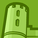# QlikView App Dev

Discussion Board for collaboration related to QlikView App Development.

Announcements
Join us for a live Q&A! September 21, 10 AM ET - Onboarding Fast in Qlik Sense SaaS! REGISTER
cancel
Showing results for
Did you mean:Contributor III

## Weighted average - Sales vs. Budget

Hi People,

Which is the best way to calculate the weighted average percentage of the following?4 RepliesMVP

@DanaL  for calculation of weighted average you need to assign the weight for each category. What are the weights for each region?Contributor III
Author

Does it mean that I have to calculate it beforehand in the script or I can do it in he chart?

What would be the final expression, assuming sales weight is X, and budget weight is Y?

Thanks!Contributor

The average is the sum of all individual observations divided by the number of observations. In contrast, the weighted average is observation multiplied by the weight and added to find a solution.

myccpayContributor III
Author

Hi @Fischer54 ,

Per table row - the calculation is correct.

So I only need it for the total line.

Is there any way to calculate the weight in the chart, or should it be done in the script?

Thanks!Community Browser